# Summand

One of the summands is 145. The second is 10 more. Determine the sum of the summands.

x =  300

### Step-by-step explanation:

$x=145+\left(145+10\right)=145+145+10=300$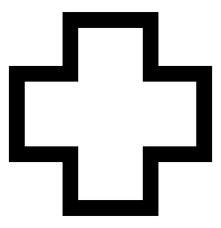Did you find an error or inaccuracy? Feel free to write us. Thank you!## Related math problems and questions:

• RemaindersIt is given a set of numbers { 170; 244; 299; 333; 351; 391; 423; 644 }. Divide this numbers by number 66 and determine set of remainders. As result write sum of this remainders.
• SummandsWe want to split the number 110 into three summands so that the first and the second summand are in the ratio 4: 5, and the third with the first are in ratio 7: 3. Calculate the smallest of the summands.
• Landlord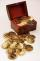Landlord had 49 ducats more than Jurošík. How many ducats Jurošík steal landlord if the Jurošík now 5 ducats more?
• Two numbersWe have two numbers. Their sum is 140. One-fifth of the first number is equal to half the second number. Determine those unknown numbers.
• ConcertOn a Concert were sold 150 tickets for CZK 360, 235 tickets for 240 CZK and 412 for 180 CZK. How much was the total revenues for tickets?
• I think numberI think number.When I add 841 to it and subtract 157, I get a number that is 22 greater than 996. What number I thinking?
• Three unknown numbers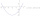The sum of of three numbers is 106. the first number is 10 more then the third. The second number is 2x the third. What are the numbers?
• The sumThe sum of the first 10 members of the arithmetic sequence is 120. What will be the sum if the difference is reduced by 2?
• Book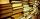Jitka read on holidays book that has 180 pages. In the first week read 45 pages. In the second week she read 15 pages more than the first week. How many pages left to read it yet?
• Identity123456789 = 100 Use only three plus or minus characters to correct previous identity/equation.
• Circles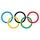For the circle c1(S1; r1=146 cm) and c2(S2; r2 = 144 cm) is distance of centers |S1S2| = 295 cm. Determine the distance between the circles.
• Quotient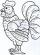Determine the quotient (q) and the remainder (r) from division numbers 100 and 8. Take the test of correctness.
• Consecutive numbersSum of ten consecutive numbers is 105. Determine these numbers (write first and last).
• Find the sumFind the sum of all natural numbers from 1 and 100, which are divisible by 2 or 5
• Bus driver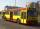The bus driver rides 174 km in the morning, afternoon rides 26 km more. How many kilometers traveled in 5 days if he rides on the same line?
• Decimal expansion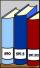Calculate: 2 . 1 + 0 . 10 + 7 . 10000 + 4 . 1000 + 6 . 100 + 0 . 100000 =
• Voting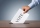Four hundred employees cast their votes in a board member election that has only two candidates. One hundred twenty people vote for candidate A, while half of the remaining voters abstain. How many votes does candidate B receive?